# 给你的 DataV 可视化大屏生成 uuid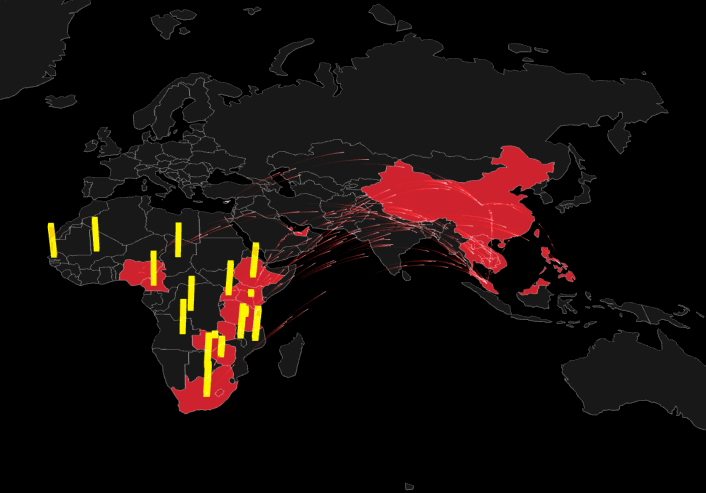## 步骤

1. 首先添加一个时间轴组件
2. 在时间轴的数据面板里填写初始化数据, 并把 value 映射我 UUID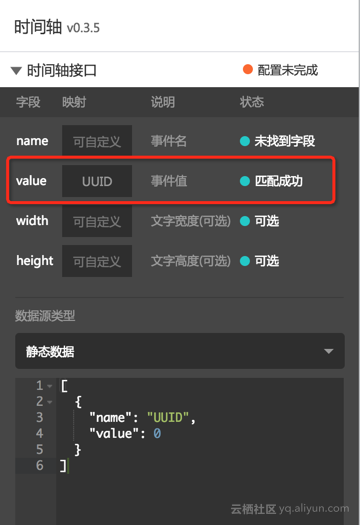3. 给时间轴组件添加过滤器，在过滤器里生成 UUID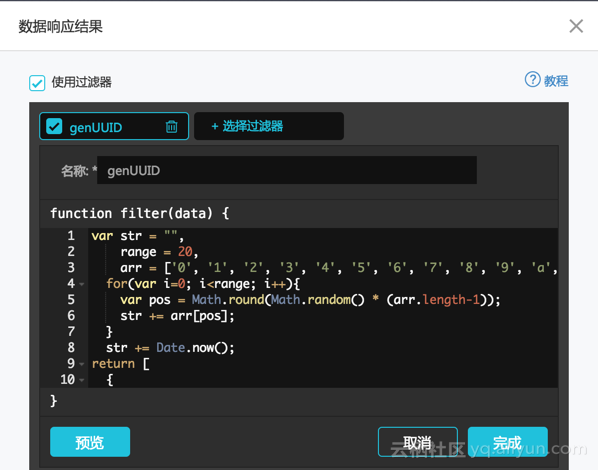var str = "",
range = 20,
arr = ['0', '1', '2', '3', '4', '5', '6', '7', '8', '9', 'a', 'b', 'c', 'd', 'e', 'f', 'g', 'h', 'i', 'j', 'k', 'l', 'm', 'n', 'o', 'p', 'q', 'r', 's', 't', 'u', 'v', 'w', 'x', 'y', 'z', 'A', 'B', 'C', 'D', 'E', 'F', 'G', 'H', 'I', 'J', 'K', 'L', 'M', 'N', 'O', 'P', 'Q', 'R', 'S', 'T', 'U', 'V', 'W', 'X', 'Y', 'Z'];
for(var i=0; i<range; i++){
var pos = Math.round(Math.random() * (arr.length-1));
str += arr[pos];
}
str += Date.now();
return [
{
UUID: str
}
];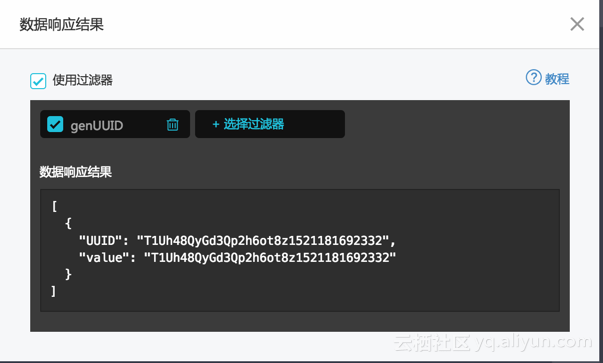1. 时间轴的样式配置里取消轮播：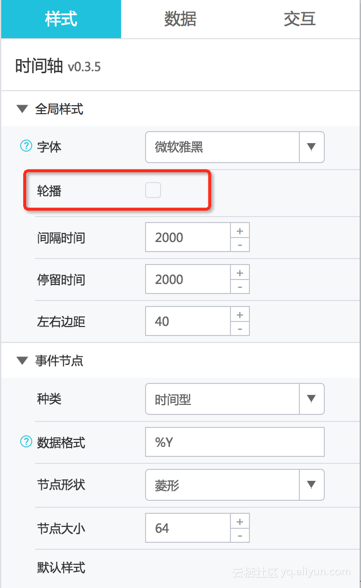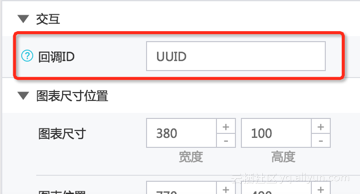1. 其他组件正常使用 API 数据源：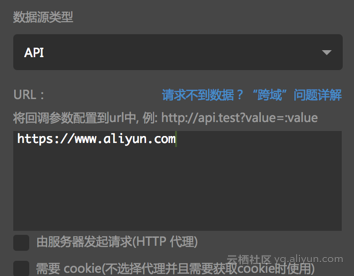2. 打开预览页面，可以看到对 API 的请求带上了 UUID 参数：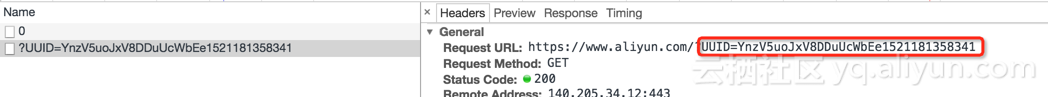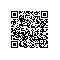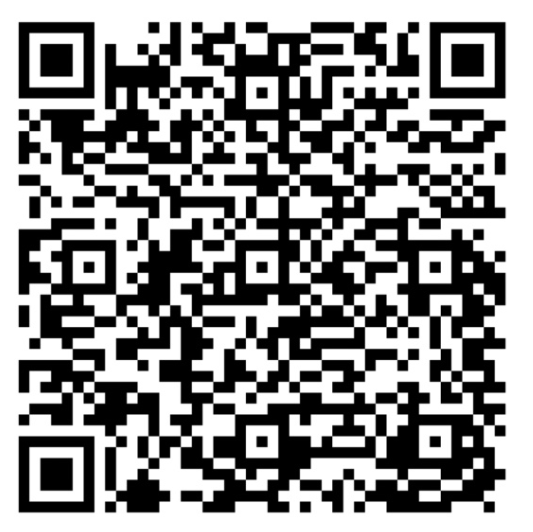使用钉钉扫一扫加入圈子
+ 订阅